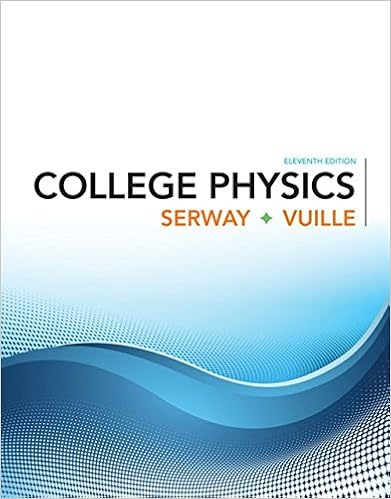A 1200 kg ferryboat is moving south at 20 ms its

• Notes
• 11
• 100% (3) 3 out of 3 people found this document helpful

This preview shows page 4 - 6 out of 11 pages.

We have textbook solutions for you!
The document you are viewing contains questions related to this textbook.The document you are viewing contains questions related to this textbook.
Chapter 7 / Exercise 7.6
College Physics
Serway/VuilleExpert Verified
20) A 1200. kg ferryboat is moving south at 20. m/s. Its momentum is A) 6.0 × 10 2 kg · m/s B) 1.7 × 10 - 3 N · s C) 24. kN · s D) 1.2 × 10 3 kg · m/s E) 2.4 × 10 3 kg · m/s
Page Ref: Sec. 6.1 21) Consider a small satellite moving in a circular orbit (radius r) about a spherical planet (mass M). The period does not depend upon
Page Ref: Sec. 7.6 22) A fan blade, whose diameter is 1.0 m, is turning with an angular velocity of 2.0 rad/s. What is the tangential velocity of a point on the tip of the blade?
Page Ref: Sec. 7.2 23) Two horizontal curves on a bobsled run are banked at the same angle, but one has twice the radius of the other. The safe speed (no friction needed to stay on the run) for the smaller radius curve is V. Therefore, the safe speed on the larger radius curve is
Page Ref: Sec. 7.3 24) The maximum speed around a level curve is 30. mi/h. What is the maximum speed around a curve with twice the radius? (Assume all other factors remain unchanged.) A) 60. mi/h B) 42. mi/h C) 45. mi/h D) 120. mi/h E) 21. mi/h
Page Ref: Sec. 7.3 25) A car, driven around a circle with constant speed, must have
Page Ref: Sec. 7.3 26) A pulsar (a rotating neutron star) emits pulses at a frequency of 0.40 kHz. The period of its rotation is
Page Ref: Sec. 7.2 4
We have textbook solutions for you!
The document you are viewing contains questions related to this textbook.The document you are viewing contains questions related to this textbook.
Chapter 7 / Exercise 7.6
College Physics
Serway/VuilleExpert Verified
27) A ball, solid cylinder, and a hollow pipe all have equal masses and radii. If the three are released simultaneously at the top of an inclined plane, which will reach the bottom first?
Page Ref: Sec. 8.4 28) A 4.0 kg mass is hung from a string which is wrapped around a cylindrical pulley (a cylindrical shell), as shown in Figure 8 - 7. Figure 8 - 7 If the mass accelerates downward at 4.90 m/s 2 , what is the mass of the pulley? A) 6.0 kg B) 4.0 kg C) 10.0 kg D) 2.0 kg E) 8.0 kg
Page Ref: Sec. 8.3 29) A pencil balanced on its tip such that it does not move
Page Ref: Sec. 8.2 30) A planet speeds up in its orbit as it gets closer to the sun because of
Page Ref: Sec. 8.5
•••